# Influence of initiation system on blast-induced ground vibration using random forest algorithm, artificial neural network, and scaled distance analysis

Influence of initiation system on blast-induced ground vibration using random forest algorithm, artificial neural network, and scaled distance analysis

Dayamoy Garai Hemant Agrawal A.K. Mishra Shankar Kumar

Coal India Limited, Kolkata 700156, India

Department of Mining Engineering, IIT(ISM), Dhanbad 826004, India

Corresponding Author Email:
hemant.ism@gmail.com
Page:
418-426
|
DOI:
https://doi.org/10.18280/mmep.050419
24 July 2018
|
Accepted:
31 August 2018
|
Published:
31 December 2018
| Citation

OPEN ACCESS

Abstract:

Explosives are used as a source of energy to break the rock mass. Majority of explosive energy is lost in the form of ground vibrations, noise, air blasts, etc. Blast-induced ground vibration is influenced by many parameters such as rock mass, explosive characteristics, blast design etc. The prediction of blast-induced ground vibration using regression analysis sometimes becomes too conservative leading difficulties in operating the mine efficiently and safely. Scaled distance approach to vibration prediction is still a very reliable predicting approach, but there are other alternative approaches which produce close results with a high value of correlation coefficient. There are modern tools for analysis and prediction which in many types of research proved to performed with more accuracy. ANN (Artificial Neural Network) is one which in fact is proved by many researchers in their papers to be an excellent prediction method of vibrations. Another method used is an ensemble learning method for classification, regression, and other tasks, that operate by constructing a multitude of decision trees at training time and outputting the class i.e. Random forest method.

In this paper, it has been tried to predict the peak particle velocities for blasts at varying distances with different initiation system using Random forest, ANN, and scaled distance regression analysis approach. The correlation coefficients for each approach for different initiation system is obtained, higher values of correlation coefficients are obtained with increase in accuracy of initiation systems due to increase in actual charge per delay during blasting. Also, it has found that the prediction is more accurate while using ANN along with digital detonators

Keywords:

ANN, random forest, blast induced ground vibration, peak particle velocity, prediction

1. Introduction

Despite the benefits of blasting as a reliable excavation technique, the main drawback of this approach lies in the induced ground vibration, which could occasionally affect structural safety during the excavation activities or it could initiate considerable rock damage and over break in underground construction. These adverse side effects of blasting may result in increased construction costs and in declining the structural stability . Having in mind such an unfavorable outcome, it is of paramount importance to adequately predict the magnitude of blast-induced vibrations to avoid the possible occurrence of rock mass failure and support damage. The standard practice uses peak particle velocity (PPV) and spectrum of excitation frequency to estimate structural responses. In practice, PPV is usually estimated using various empirical relations, which are of significant interests for field engineers, since they enable them to predict the maximum ground vibration depending on the maximum charge per delay and distance from the blasting source . In effect, these empirical equations represent various nonlinear relations between the values of PPV on one side, and scaled distance (distance from the explosion charge normalized by the amount of explosive used) , on the other side. Indeed, practical application has shown that this so-called ''conventional predictors'' give reasonable predicting values for engineering purposes. However, these empirical relations are typically developed for specific site conditions with particular local geological settings and field morphology and cannot predict PPV value with satisfying accuracy at other blasting locations, mainly due to heterogeneous and anisotropic rock mass properties. This further implies that the suggested ground motion relations could not give reliable estimation of PPV, mainly due to fact that these models are approximate, treating blast-induced ground vibrations dependence only on maximum charge per delay or distance to the blasting source, neglecting a number of other influential parameters, like total charge, stemming, hole depth, physical and mechanical properties of rock mass or explosive characteristics. Since the number of affecting parameters is large and the relations among them could be very complicated and often unknown, empirical methods may not always be suitable for accurate prediction of PPV . Numerical methods are used now a day to predict the vibration produced by blasting by many researchers .

As far as numerical methods are concerned, different numerical methods like Artificial neural network [6-8], Support vector machine [9-11], Adaptive neuro-fuzzy interface system(ANFIS) [12-13], Monte Carlo technique  and many more such methods have been used to predict and control blast induced ground vibration. All these methods use multiple input parameters to get the most suitable relation between input and output parameters. More advanced numerical approaches are related to the analysis of block systems . In such models, systems of simultaneous equations are formulated and solved minimizing the energy of the system to bring the system into equilibrium. , Khandelwal et al. and Khandelwal and Singh  made use of such useful factors as the rock type, blasting pattern, and explosive type (in addition to factors like the maximum weight of the explosive per delay and the distance between the measuring point and the center of the explosion block) as the input parameters to the ANN to determine the PPV and compared their results with those of similar methods available at the time. , studied the effects of the number of blast holes rows on the PPV using the ANN. Bahadori et al. employed GA to improve the correlation coefficient for empirical relations used in the prediction of the PPV. Utilizing the GA Hybrid method, Soltani et al.  also predicted the allowable charge weight per delay in blasting operations in the construction of underground structures in Gotvand Olya Dam. Longjun Dong et al.  has used random forest algorithm and support vector machine for predicting blast-induced ground vibration.

Here in this paper out of different numerical methods, random forest algorithm, Artificial neural network (ANN) were taken in consideration with the Scaled distance regression analysis approach. All these three methods are applied to predict the blast-induced ground vibration in terms of peak particle velocities. The correlation coefficients for each approach for different initiation system is obtained, based on the values of the correlation coefficients for different initiating system it is found that the use of most precise digital/ electronic detonators in blasting with ANN as predicting tool is most suitable to enhance safety in blasting operations.

2. Initiation Systems Used

The most frequently used initiation systems were considered in this study. They were namely Detonating cord with cord relay, Non-electric (NONEL) detonators, Electronic detonators Initiation system:

2.1 Detonating cord with cord relay

Detonating cord is a thin, flexible plastic tube usually filled with pentaerythritol tetranitrate (PETN). With the PETN exploding at a rate of approximately 6400 m/s, any common length of detonation cord appears to explode instantaneously. It is a high-speed fuse which explodes, rather than burns, and is suitable for detonating high explosives. The pyrotechnic detonators used with detonating cord shows a high cap scatter percentage and also high blast-induced ground vibrations.

2.2 Non-electric (nonel) initiation system

A non-electric detonator is a shock tube detonator designed to initiate explosions, generally for the the demolition of buildings and use in the blasting of rock in mines and quarries. Instead of electric wires, a hollow plastic tube delivers the firing impulse to the detonator, making it immune to most of the hazards associated with stray electric current. It consists of a small diameter, three-layer plastic tube coated on the innermost wall with a reactive, explosive compound, which, when ignited, propagates a low energy signal, similar to a dust explosion. The reaction travels at approximately 6,500 ft/s (2,000 m/s) along the length of the tubing with a minimal disturbance outside of the tube. The design of non-electric detonators incorporates a patented technology, including the Cushion Disk (CD) and Delay Ignition Buffer (DIB) to provide reliability and accuracy in all blasting applications. Non-electric detonators were invented by the Swedish company Nitro Nobel in the 1960s and 1970s, under the leadership of Per-Anders Persson, and launched to the demolitions market in 1973. Nonel is a contraction of "Non-electric detonators". The shock wave travels inside the shock tube initiates the pyrotechnic detonator. The pyrotechnic delay detonators with NONEL initiation system show a significant cap scatter.

2.3 Electronic initiation system

In mining, electronic detonators have a better precision for delays. Electronic detonators are designed to provide the precise control necessary to produce accurate and consistent blasting results in a variety of blasting applications in the mining, quarrying, and construction industries. Electronic detonators may be programmed in 1-millisecond increments from 1 millisecond to 10,000 milliseconds using the dedicated programming device called the logger. Due to high precision in its timing the cap scatter is too low .

3. Random Forest Algorithm

Random forest algorithm is a supervised classification algorithm. As the name suggests, this algorithm creates the forest with a number of trees. In general, the more trees in the forest, the more robust the forest looks like. In the same way in the random forest classifier, the higher the number of trees in the forest gives the high accuracy results. Decision tree concept is more to the rule-based system. Given the training dataset with targets and features, the decision tree algorithm will come up with some set of rules (Figure 1). The same set rules can be used to perform the prediction on the test dataset .

• The same random forest algorithm or the random forest classifier can use for both classification and the regression task.
• Random forest classifier will handle the missing values.
• When we have more trees in the forest, random forest classifier won’t overfit the model.
• Can model the random forest classifier for categorical values also.

## 1.jpg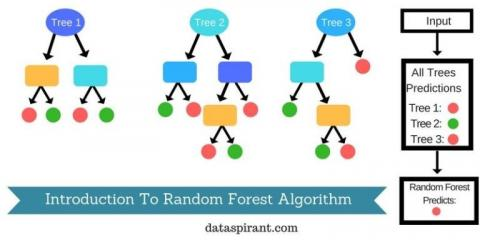Figure 1. Pictorial representation of random forest algorithm

4. Artificial Neural Network

Artificial neural networks can be used to extract patterns and detect trends that are too complex to be noticed by humans or computer techniques. They can be used to provide projections of given new situations and answer "what if" questions. ANNs have some other advantages, which include:

• Adaptive Learning, which is the ability to do tasks, based on given data used for training.
• Self-Organization in which ANNs can create their organization or representation of the information during learning time.
• Real-Time Operation in which ANNs computations may be carried out in parallel.
• Fault Tolerances in which some network capabilities may be retained even with major network damage; as a partial destruction of a network leads to the corresponding degradation of performance.

There is a broad range of applications that can be found for artificial neural networks (Figure 2). The applications are expanding because artificial neural networks are good at solving problems, not just in

engineering, science, and mathematics, but in medicine, business, finance, and literature as well. Their application to a wide variety of problems in many fields makes them very attractive. Also, faster computers and faster algorithms have made it possible to use artificial neural networks to solve complex industrial problems that formerly required too much computation .

## 2.jpg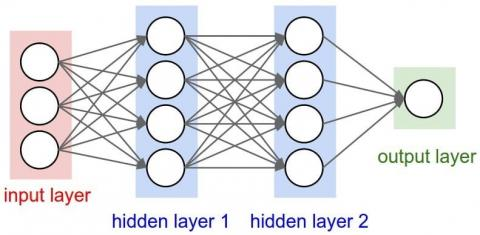Figure 2. Representation of Artificial neural network (ANN) process

In this the multiple input parameters were taken for ANN and Random Forest analysis with a single output. While using ANN, 10 numbers of hidden neurons were selected.Where in random forest 100 number of iterations were performed. The input and output parameters taken for analysis are shown in Table 1 below.

Table 1. Input and output parameters taken for ANN and Random forest analysis

 Input parameters are in ANN, and Random forest analysis are Output Parameter No. of rounds No. of observations The depth of drill holes (m) The diameter of the hole (mm) Burden (m) Spacing (m) Maximum charge/delay (kg) Maximum charge/round (kg) Peak Particle velocity (mm/s)
5. Scaled Distance Regression Analysis

Scaled Distance regression analysis approach was given by USBM . The scaled distance is a concept put forward by using the amount of explosive energy in shock and seismic waves, and the effects by distance. The scaled distance (SD) is derived by combining the distance between the source and measurement points, and the maximum charge per delay. This scaled distance is defined by the equation below:

$S D=D / \sqrt{W}_{d}$   (1)

where SD is the scaled distance (m/kg1/2), D is the absolute distance between the shot and the station (m), and Wd is the maximum explosive charge per delay (kg).

The peak level of ground motion at any given point is inversely proportional to the square of the distance from the shot point .  The peak particle velocity (PPV) is given by the following equation:

$P P V=K \times(S D)^{n}$   (2)

where K and n are site/ geological constant factors. The site factors are determined from a logarithmic plot of peak particle velocity (PPV) versus scaled distance (SD). The straight-line best representing the data has a negative slope n and an intercept K.

6. Methodology

In this paper the blast-induced ground vibration data was collected with different initiation system (Electronic initiation, Nonel and Detonating cord with cord relay), actual peak particle velocity (PPV) and blast design parameters were recorded for each. After getting the actual values and blast design parameters, the data collected was processed individually for each initiation system using the random forest algorithm and Artificial Neural Network (ANN). The input data used are blast design parameters, the distance at which the vibration was recorded and maximum charge per delay to get the output of actual PPV values collected. The closest relation between input data and output data (Table 1) were generated with a minimum possible error with each approach (random forest and ANN), and values of PPV were predicted with its correlation coefficient for each initiating system. Also, the scale distance regression analysis is done with different initiations system, and correlation coefficient for the curve is calculated. After applying each approach (Random forest, ANN, and Scaled distance) for different initiation system, the values of correlation coefficient were analyzed to find the effect of accuracy of initiation systems on blast-induced ground vibrations.

6.1 Data collection

The study site (i.e. an opencast (OC) coal mine) is located in an part of Jharia Coalfield in Dhanbad district of Jharkhand (Figure 3). The OC Mine is being worked using shovel-dumper combination for removal of (OB), extraction & transportation of coal. The rock strata in the mine area belong to Barakar Formation of Lower Gondwana group under cover of soil, alluvium and sandy soil.

The blast-induced ground vibration was measured by three numbers of MINIMATE PLUS (Instantel Inc. Canada) vibration monitors. These instruments are a microprocessor-based unit having tri-axial transducers. The instrument measures Peak Particle Velocity (PPV) in three mutually perpendicular directions, i.e. longitudinal, transversal and vertical along with respective frequencies and amplitude.

## 3.jpgFigure 3. Google Earth image of data collection site

The vibration data for each initiation system, i.e. Electronic initiation system, Non-electric (NONEL) and detonating cord with cord relay were collected. Details of vibration data collected with each initiating system are shown in Table 2 below:

Table 2. Details of vibration data collected at an opencast coal mine

 Details of Blast Initiation System NONEL Electronic Delay Detonator Detonating Cord No. of rounds 10 14 10 No. of observations 36 54 38 The depth of drill holes (m) 4.4 – 5.9 m 4.3 – 6.4 m 3.9 – 6.1 m The diameter of hole (mm) 160 160 160 Burden (m) 3 3 3 Spacing (m) 3.5 3.5 3.5 Explosive charge/hole (kg) 27.15 – 42.15 30.15 – 60.15 20.15 – 50.15 Maximum charge/delay (kg) 42.15 60.15 50.15 Maximum charge/round (kg) 1634.2 1986.75 1949.15
7. Analysis

As discussed above methodology the data collected is processed using Random forest algorithm, Artificial neural network (ANN) and Scaled distance regression analysis approach for each initiating system.

7.1 Detonating cord with cord relay

The vibration data collected were analyzed using the forest, ANN and scaled distance and predicted the of PPV and Correlation coefficient is calculated as shown in Table 3. Also, the analysis using scaled distance, ANN, the forest is shown in Figure 4, Figure 5 and Figure 6 respectively. Figure 4 is a plot between PPV and Scaled Distance as per scaled distance regression analysis which shows the power relation with correlations coefficient of 0.9429. Figure 5 is the representation of training, testing and validation of data using ANN with their correlation coefficients. Figure 6 is the report of random forest algorithms used for prediction of PPV with the collected data od PPV using D-cord.

## 4.pngFigure 4. Scaled distance regression analysis equation using the Detonating cord

## 5.jpgFigure 5. Artificial neural network (ANN) analysis for Detonating cord initiation system

## 6.jpg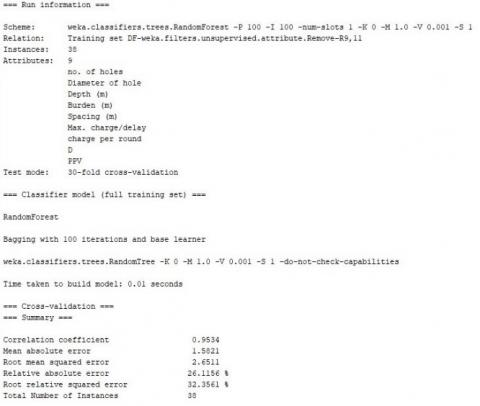Figure 6. Random forest analysis for detonating cord initiation system

The predicted values of PPV using Scaled distance, ANN and random forest algorithm and actual values of PPV recorded for blasts using detonating cord with cord relay is plotted on a same curve in Figure 7.

## 7.png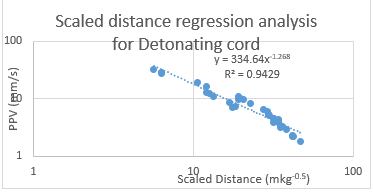Figure 7. Comparison curve b/w Actual PPV and RF, ANN, Scaled Distance predicted value for detonating cord

Table 3. The Predicted value of PPV using Random forest, ANN and Scaled distance for detonating cord

 PPV Actual Predicted PPV Using Random Forest (R2 = 0.9534) Using ANN (0.9659) Using Scaled distance (R2 =0.9429) 1.769 3.03 1.787914 2.564921 2.174 2.851 2.072367 2.894409 2.203 2.888 2.177766 2.956755 2.25 2.653 1.933229 2.956755 2.924 3.582 2.785779 3.308113 3.205 4.488 3.287279 3.647218 3.304 3.929 3.160113 3.556777 3.469 4.104 3.405996 3.690769 3.814 3.583 3.748549 3.840978 3.829 4.663 4.134037 4.146625 4.028 4.516 5.222822 4.131416 4.062 4.07 3.825649 3.7928 4.325 3.932 3.825649 3.7928 4.492 4.688 4.134037 4.146625 4.537 4.24 4.324689 4.168523 4.581 4.062 4.324689 4.168523 5.197 6.561 4.893068 4.500235 5.287 5.241 5.293765 4.549625 5.293 5.29 5.159811 4.549625 5.872 4.745 5.596651 4.690787 6.56 5.043 6.098105 4.997879 6.92 8.787 7.123752 8.783503 7.364 9.142 6.613665 8.357367 8.131 7.994 6.979745 6.303098 8.655 9.729 12.70883 9.250129 9.563 7.255 9.246324 7.276709 9.838 8.649 10.32998 7.966846 10.29 9.352 10.14682 7.607803 10.85 13.224 10.42117 7.966846 11.13 11.9 10.73303 12.4393 12.35 11.162 11.42628 13.32202 12.81 20.314 18.07711 14.32341 16.59 13.752 18.19589 14.32341 19.46 15.931 20.11885 16.78696 27.29 26.063 26.18906 32.08309 28.28 21.503 26.53367 32.08309 28.84 25.109 26.18906 32.08309 32.26 21.355 27.61853 37.25097

1. NONEL INITIATING SYSTEM

The vibration data collected using NONEL initiating system were analyzed using the forest, ANN and scaled distance and predicted of PPV and Correlation coefficient is calculated as shown in Table 4. Also, the analysis using scaled distance, ANN forest is shown in Figure 8, Figure 9 and Figure 10 respectively. Figure 8 is a plot between PPV and Scaled Distance as per scaled distance regression analysis which shows the power relation with correlations coefficient of 0.942. Figure 9 is the representation of training, testing and validation of data using ANN with their correlation coefficients. Figure 10 is the report of random forest algorithms used for prediction of PPV with the collected data od PPV using NONEL initiating system.

Table 4. Predicted value of PPV using Random forest, ANN and Scaled distance for Nonel initiating system

 PPV Actual Predicted PPV Using Random Forest (R2 = 0.9568) Using ANN (R2=0.966) Using Scaled distance (R2 =0.942) 2.275 3.024 2.439494 2.711871 2.376 3.203 2.862266 2.781619 2.492 3.005 2.817177 2.485645 2.53 2.967 2.483618 2.781619 2.658 2.799 2.907473 2.538706 2.768 2.839 2.452363 2.781619 2.843 2.861 2.552501 2.854828 2.965 3.005 2.686117 2.931754 2.973 2.85 2.997327 2.593942 3.016 3.009 2.899065 3.097929 3.061 2.889 3.086917 2.651489 3.238 3.219 3.011483 3.187838 3.336 3.199 3.11523 3.282795 3.522 3.619 3.27366 3.438851 3.637 3.318 3.238019 3.383228 3.65 4.015 3.591371 3.783643 4.006 4.566 4.014179 4.528679 4.127 4.102 4.054218 4.132002 4.176 4.077 4.220859 4.287975 4.229 4.076 4.404289 4.455429 4.301 4.389 4.428307 4.711864 4.355 4.087 4.831371 4.830123 4.529 5.333 5.25449 5.123437 5.039 6.033 5.622193 5.355612 5.718 4.171 6.22363 5.268986 5.842 6.29 5.662265 5.190824 5.996 6.335 6.142596 5.458222 6.142 6.969 6.726847 6.780088 6.361 6.242 6.142596 5.458222 7.113 6.744 6.380642 5.884761 7.589 6.328 6.896128 6.187905 7.925 6.559 8.19112 7.302805 7.972 7.528 8.663334 7.762889 9.341 8.487 9.184069 10.63985 9.808 8.159 9.184069 10.63985

## 8.png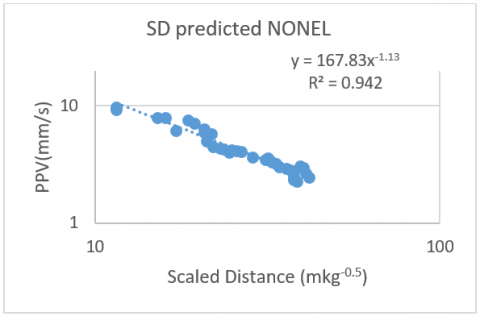Figure 8. Scaled distance regression analysis equation using Nonel initiating system

## 9.jpg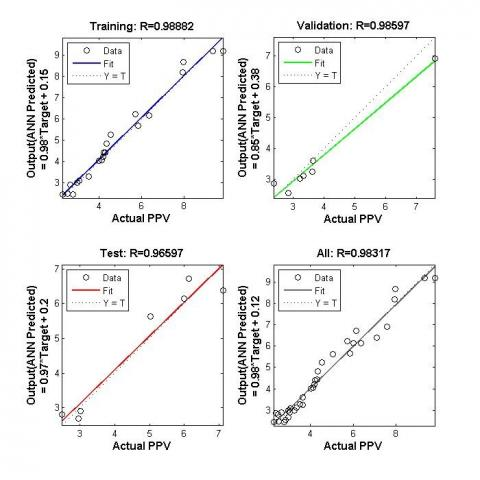Figure 9. Artificial neural network (ANN) analysis for Nonel initiation system

## 10.jpg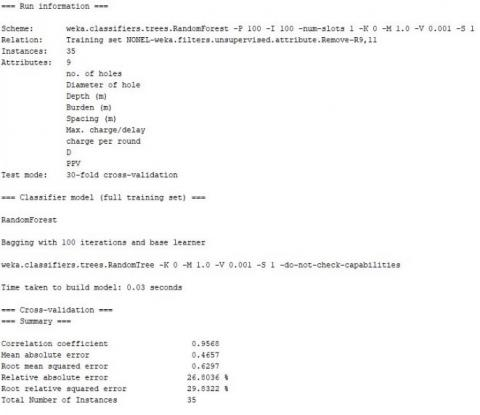Figure 10. Random forest analysis for NONEL initiation system

The predicted values of PPV using Scaled distance, ANN and random forest algorithm and actual values of PPV recorded for blasts using NONEL initiating system is plotted on a same curve in Figure 11.

## 11.png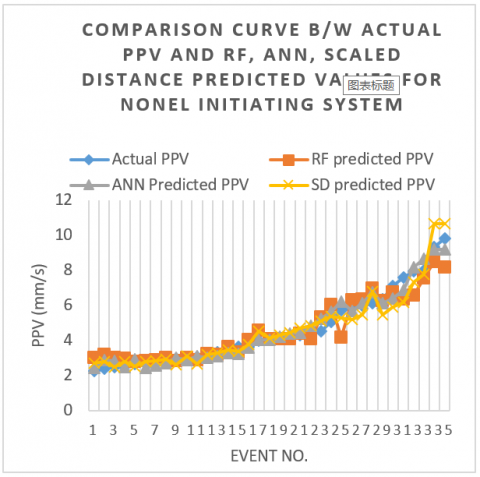Figure 11. Comparison curve b/w Actual PPV and RF, ANN, Scaled Distance predicted value for NONEL initiating system

1. ELECTRONIC INITIATION SYSTEM

The vibration data collected using electronic initiating system were analyzed using forest, ANN and scaled distance and predicted of PPV and Correlation coefficient is calculated as shown in Table 5. Also, the analysis using scaled distance, ANN forest is shown in figure 12, figure 13 and figure 14 respectively. Figure 12 is a plot between PPV and Scaled Distance as per scaled distance regression analysis which shows the power relation with correlations coefficient of 0.9418. Figure 13 is the representation of training, testing and validation of data using ANN with their correlation coefficients. Figure 14 is the report of random forest algorithms used for prediction of PPV with the collected data od PPV using electronic initiations system.

## 12.jpgFigure 12. Scaled distance regression analysis equation using the Electronic initiating system

## 13.png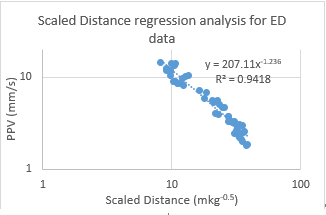Figure 13. Artificial neural network (ANN) analysis for Electronic initiation system

Table 5. Predicted value of PPV using Random forest, ANN and Scaled distance for Electronic initiating system

 PPV Actual Predicted PPV Using RF (R2 = 0.9793) Using ANN (R2= 0.980) Using Scaled distance (R2=0.9418) 1.814 2.719 1.863577 2.313641 1.836 2.588 1.893469 2.248813 1.98 2.682 1.808023 2.559409 2.106 2.24 2.41516 2.593936 2.195 2.589 2.355597 2.700735 2.311 2.909 2.593305 2.75085 2.376 2.343 2.279329 2.628355 2.413 2.897 2.460693 3.056178 2.529 2.913 2.738952 2.468794 2.555 3.064 2.67389 2.410246 2.63 2.611 2.499497 2.896252 2.843 2.521 2.590475 2.821858 2.981 2.49 2.557442 2.618138 2.992 2.779 3.10345 2.529966 3.069 2.81 3.596864 2.731044 3.116 3.364 3.378178 3.048418 3.253 3.418 3.463081 3.13838 3.263 3.126 2.666989 3.030064 3.277 3.328 2.590428 3.42885 3.319 3.495 2.450034 3.328229 3.326 3.453 3.485032 3.233261 3.72 3.109 2.471426 3.42885 3.772 3.352 3.628103 3.439424 3.923 5.192 4.34306 4.280846 4.066 4.847 4.750226 4.64867 4.697 4.423 4.350185 3.797097 4.713 4.675 4.36847 3.931638 5.101 4.971 4.918485 4.228228 5.348 5.212 5.577978 4.854733 5.445 4.829 5.14294 4.39214 5.483 4.954 5.429902 4.64867 5.561 5.208 5.193582 4.39214 5.608 5 5.886405 4.960411 5.849 6.318 6.185736 5.873697 6.854 4.466 6.510324 5.584686 7.209 6.166 7.495926 6.540609 8.149 8.788 8.016308 9.36057 8.581 8.464 8.501341 10.00748 8.649 8.739 9.000617 10.74006 8.966 10.944 9.992867 11.20451 8.986 8.811 9.52164 11.57576 9.789 10.099 9.575193 9.598574 10.29 9.273 9.431857 8.943855 10.35 9.549 9.170657 8.365697 10.54 11.476 10.83264 12.53698 11.57 10.66 10.83264 12.53698 11.71 11.985 11.84679 13.65298 11.87 12.95 13.54578 12.53195 12.36 11.56 11.84679 13.65298 13.49 12.423 12.75992 11.43506 13.91 13.047 12.75871 11.43506 14.12 11.778 14.1605 11.20451 14.2 11.85 14.1847 12.2019 14.45 12.603 14.80748 15.40605

## 14.jpgFigure 14. Random Forest (RF) analysis for Electronic initiation system

The predicted values of PPV using Scaled distance, ANN and random forest algorithm and actual values of PPV recorded for blasts using electronic initiating system is plotted on a same curve in Figure 15.

## 15.pngFigure 15. Comparison curve b/w Actual PPV and RF, ANN, Scaled Distance predicted value for Electronic initiating system

8. Comparison of Correlation Coefficients

The analysis was made for different initiating system, i.e. Detonating cord, Nonel and Electronic initiation system using forest, ANN and Scaled distance regression analysis and the correlation coefficients obtained and presented below in Table 6 and Figure 16 below.

Table 6. Summary of correlation coefficient obtained using Scaled distance (SD), ANN and Random forest (RF) methods for different initiating system

 Initiating System R2 value with RF R2 value with ANN R2 value with SD ED 0.9793 0.980 0.9418 NONEL 0.9568 0.966 0.9429 DF 0.9534 0.9659 0.942

## 16.png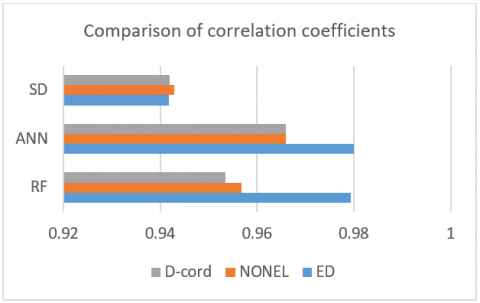Figure 16. Values of correlation coefficients obtained with different analysis for different initiation system

9. Discussion

With the correlation coefficients obtained between predicted and actual PPV value with Random forest, ANN and scaled distance regression analysis while using different initiation systems, it can be seen that the highest value of correlation coefficient is observed with ANN analysis method. Whereas least value of correlation coefficient is obtained by Scaled distance regression analysis approach. A significant value of correlation coefficient which is higher than Scaled distance regression analysis approach is found using Random forest algorithm.

Further, it can be seen that the highest value of correlation coefficient has been obtained with the Electronic initiation system, and with Detonating cord. This implies that the relation generated by the Random forest method, ANN and Scaled distance method between the input parameters (blast design parameters, the distance at which vibration reading was recorded and maximum charge per delay) and an output parameter (vibration in PPV) is more accurate in case of an Electronic initiation system. This may be attributed to the fact that the measured charge per delay might have been different than the actual charge per delay experienced during blasting due to cap scattering or inaccuracies in firing time of detonators. The maximum charge per delay is more accurate or the actual charge per delay in case of Electronic initiation system. Electronic initiation system is accurate and each hole blasts at their designated time due to very less cap scattering (±0.05%). The value of charge per delay considered for NONEL and Detonating cord initiation system might have been different than the actual charge per delay as the higher cap scattering results overlapping of detonation of holes.

10. Conclusion

The study conducted in this paper has indicated the following useful inferences.

• Out of the three methods used in this paper for prediction of blast-induced ground vibration, Artificial neural network (ANN) has predicted the most accurate value with highest correlation coefficients. While Random forest algorithm predicted the values with lower correlation coefficient than ANN but higher than Scaled distance regression approach. Which makes ANN the preferred tool (Among the three tools used) for predicting blast-induced ground vibrations in blasting.
• The highest value of correlation coefficient with all three methods used for prediction is obtained with Electronic initiation system. This indicates that due to the accuracy of Electronic initiation system the actual charge per delay is accurate which helps in the precise prediction of blast-induced ground vibration.
• On the basis of the conducted study, it may be concluded that for calculating the maximum charge per delay in controlled blasting operation, electronic detonators with ANN predictor may be used for precise prediction of blast-induced ground vibration in control blasting over the random forest and scaled distance regression analysis. Which will be a great help to mine operators in the conduction of controlled blasting near habitation.
References

 Agrawal H, Mishra AK. (2018). A study on influence of density and viscosity of emulsion explosive on its detonation velocity. Model. Meas. Control C 78(03): 316–336. http://dx.doi.org/10.18280/mmc_c.780305

 Kumar R, Choudhury D, Bhargava K. (2016). Determination of blast-induced ground vibration equations for rocks using mechanical and geological properties. J. Rock Mech. Geotech. Eng. 8(3): 341–349. http://dx.doi.org/10.1016/j.jrmge.2015.10.009

 Duvall WI, Fogelson DE. (1962). Review of criteria for estimating damage to residences from blasting vibrations. US Department of the Interior, Bureau of Mines.

 Sayadi A, Monjezi M, Talebi N, Khandelwal M. (2013). A comparative study on the application of various artificial neural networks to simultaneous prediction of rock fragmentation and backbreak. J. Rock Mech. Geotech. Eng. 5(4): 318–324. http://dx.doi.org/10.1016/j.jrmge.2013.05.007

 Agrawal AKMH. (2018). Probabilistic analysis on scattering effect of initiation systems and concept of modified charge per delay for prediction of blast induced ground vibrations. Measurement 130: 306–317. http://dx.doi.org/10.1016/j.measurement.2018.08.032

 Khandelwal M, Singh TN. (2009). Prediction of blast-induced ground vibration using artificial neural network. Int. J. Rock Mech. Min. Sci. 46(7): 1214–1222. http://dx.doi.org/10.1016/j.ijrmms.2009.03.004

 Singh TN, Singh V. (2005). An intelligent approach to prediction and control ground vibration in mines. Geotech. Geol. Eng. 23(3): 249–262. http://dx.doi.org/10.1007/s10706-004-7068-x

 Bakhshandeh Amnieh H, Bahadori M. (2014). Safe vibrations of spilling basin explosions at ‘Gotvand Olya Dam’ using artificial neural network. Arch. Min. Sci. 59(4): 1087–1096.

 Khandelwal M, Kankar PK, Harsha SP. (2010). Evaluation and prediction of blast induced ground vibration using support vector machine. Min. Sci. Technol. 20(1): 64–70. http://dx.doi.org/10.1016/S1674-5264(09)60162-9

 Verma AK, Singh TN. (2013). Comparative study of cognitive systems for ground vibration measurements. Neural Comput. Appl. 22(1): 341–350. http://dx.doi.org/10.1007/s00521-012-0845-1

 Rao YS. (2012). Prediction of ground vibrations in opencast mine using nonlinear regression analysis. Int. J. Eng. Sci. Technol. 4(9).

 Singh TN, Dontha LK, Bhardwaj V. (2008). Study into blast vibration and frequency using ANFIS and MVRA. Min. Technol. 117(3): 116–121. http://dx.doi.org/10.1179/037178409X405741

 Trivedi R, Singh TN, Mudgal K, Gupta N. (2014). Application of artificial neural network for performance evaluation of vertical axis wind turbine rotor. Int. J. Ambient Energy 3(5): 564–574.

 Blair DP. (2004). Charge weight scaling laws and the superposition of blast vibration waves. Fragblast 8(4): 221–239. http://dx.doi.org/10.1080/13855140412331291610

 Mortazavi A, Katsabanis PD. (2001). Modelling burden size and strata dip effects on the surface blasting process. Int. J. Rock Mech. Min. Sci. 38(4): 481–498. http://dx.doi.org/10.1016/S1365-1609(01)00015-6

 Kostić S, Vasović N, Franović I, Samčović A, Todorović K. (2014). Assessment of blast induced ground vibrations by artificial neural network. In Neural Network Applications in Electrical Engineering (NEUREL), pp. 55–60. http://dx.doi.org/10.1109/NEUREL.2014.7011458

 Khandelwal M, Singh TN. (2007). Evaluation of blast-induced ground vibration predictors. Soil Dyn. Earthq. Eng. 27(2): 116–125. http://dx.doi.org/10.1016/j.soildyn.2006.06.004

 Dong L, Li X, Xu M, Li Q. (2011). Comparisons of random forest and Support Vector Machine for predicting blasting vibration characteristic parameters. Procedia Eng. 26: 1772–1781.

 Agrawal H, Mishra AK. (2018). Evaluation of initiating system by measurement of seismic energy dissipation in surface blasting. Arab. J. Geosci. 11(13): 345. http://dx.doi.org/10.1007/s12517-018-3683-3

  Liaw A, Wiener M. (2002). Classification and Regression by randomForest. R News 2(December): 18–22.

 Tawadrous A. (2006). Evaluation of artificial neural networks as a reliable tool in blast design. In Proceedings of the Annual Conference on Explosives and Blasting Technique 32(1): 71.

 Physicist S, Duvall WI, Fogelson DE. (2000). Review of Criteria for Estimating Damage To, no. April 1961, pp. 1-16.